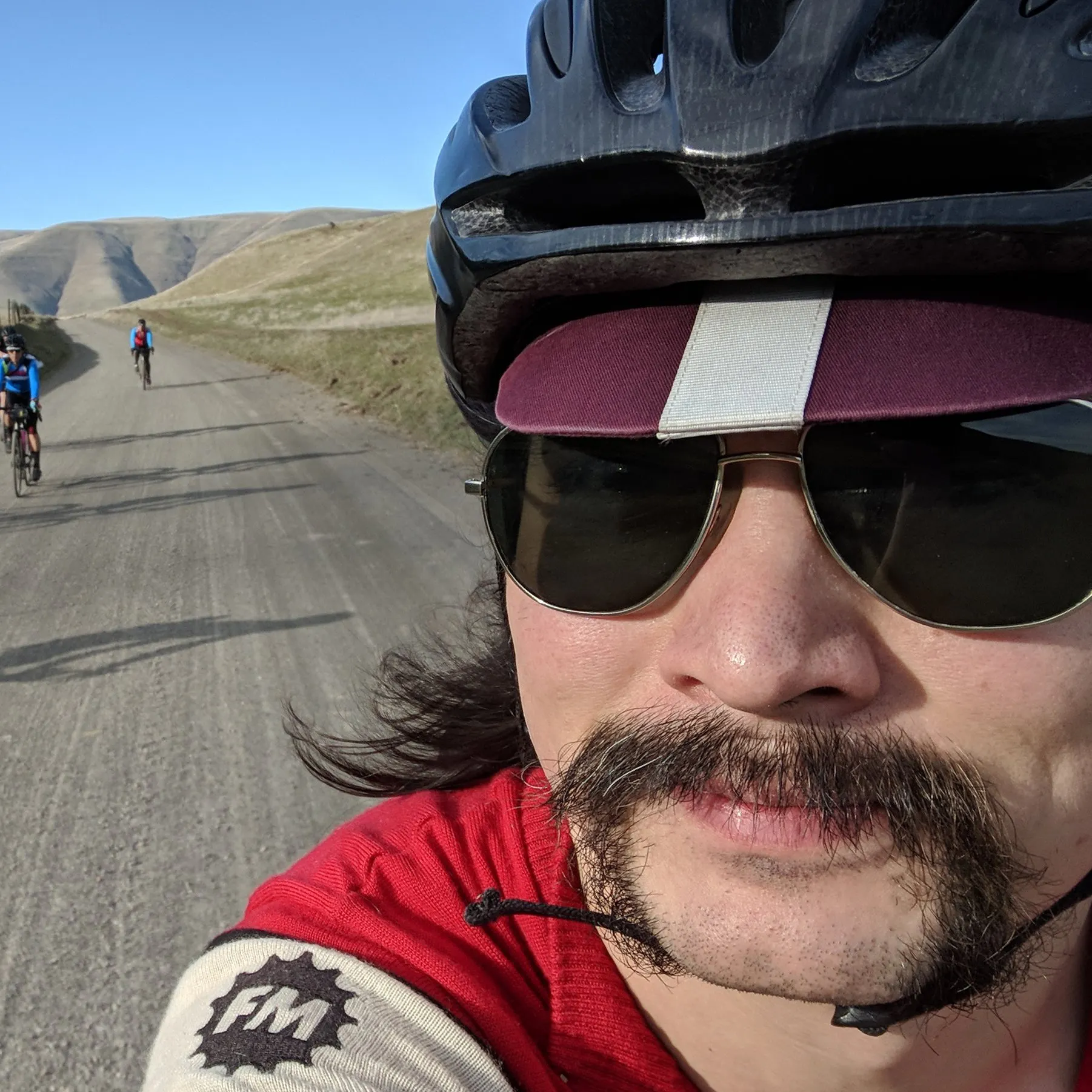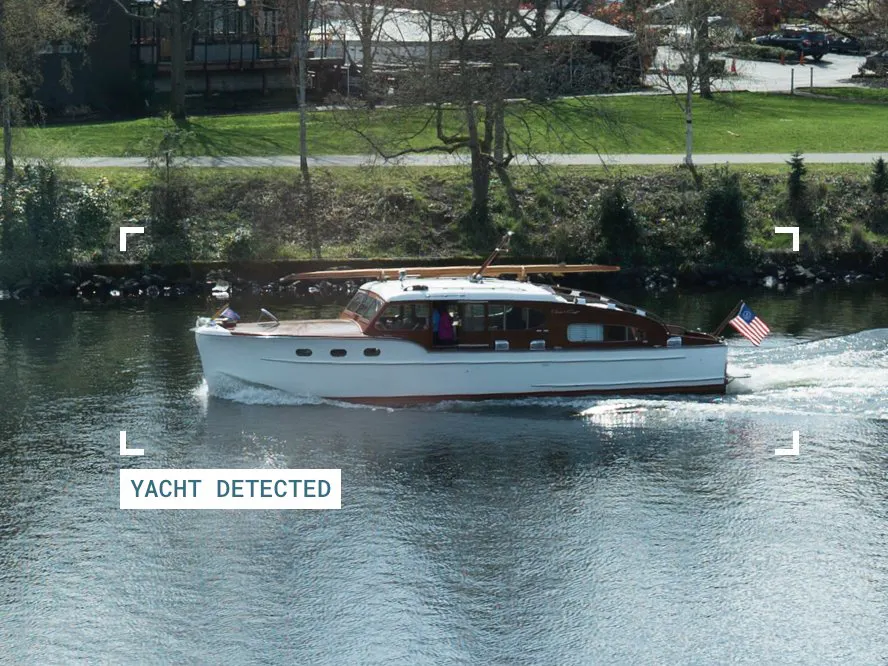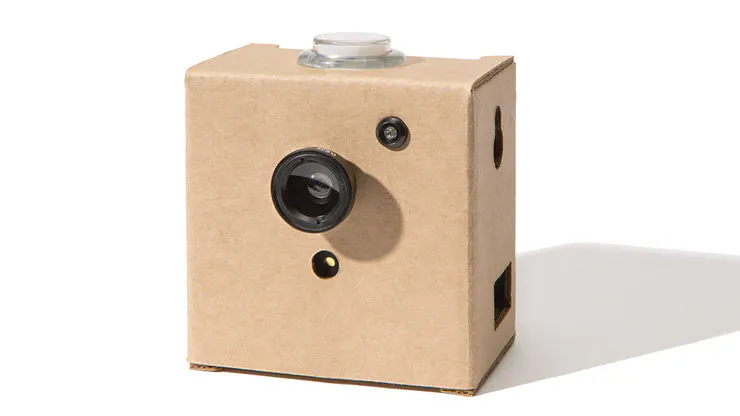Published

# Yacht TV

Automatically detect boats and save video clips as they pass. Based on a Google AIY Vision Kit.

IntermediateFull instructions provided2 hours2,242## Things used in this project

### Hardware components×1

## Code

### Video Detection Capture

Python
A Python script for use on the AIY Vision Kit. Insert it into the vision examples directory, then run from the command line. See the --help option for more info.
```#!/usr/bin/env python3
#
# you may not use this file except in compliance with the License.
# You may obtain a copy of the License at
#
#
# Unless required by applicable law or agreed to in writing, software
# WITHOUT WARRANTIES OR CONDITIONS OF ANY KIND, either express or implied.
# See the License for the specific language governing permissions and
"""Video capture by class detection demo.
This script continuously monitors the Raspberry Camera and tries to detect
instances of a set of specified classes/categories. When on is detected a
short video file is written capturing briefly before and after the capture.
Example usage:
python video_capture.py -c boat_classes.txt --out_dir my_captures/
The file boat_classes.txt contains the desired set of classes to look for.
It is simply a text file containing one class per line:
catamaran
container ship/containership/container vessel
lifeboat
speedboat
pirate/pirate ship
submarine/pigboat/sub/U-boat
fireboat
A full list of possible categories can be obtained here:
<url>
"""
import argparse
import io
import numpy as np
import os
import picamera
import pickle
import sys
import time
from PIL import Image
from aiy.vision.inference import ImageInference
from aiy.vision.models import image_classification
def crop_parameters(im, range_x=[0, 1], range_y=[0, 1]):
"""Yields crop parameters for the given x- and y-ranges"""
size = np.array(im.size).astype(np.int)
crop_size = (size / 4).astype(np.int)
step = (crop_size / 2).astype(np.int)
x_start = int(range_x * size)
x_end = int(range_x * size - crop_size) + 1
y_start = int(range_y * size)
y_end = int(range_y * size - crop_size) + 1
for y in range(y_start, y_end, step):
for x in range(x_start, x_end, step):
yield (x, y, x + step * 2, y + step * 2)
debug_idx = 0
def debug_output(image, debug_data, out_dir, filename=None):
"""Outputs debug output if --debug is specified."""
global debug_idx
if debug_idx == 0:
for filepath in [f for f in os.listdir(out_dir) if f.startswith('image_')]:
try:
path_idx = int(filepath[6:12]) + 1
debug_idx = max(debug_idx, int(filepath[6:12]) + 1)
except:
pass
print('debug_idx:', debug_idx)
if filename == None:
output_path = os.path.join(out_dir, 'image_%06d.jpg' % (debug_idx))
debug_idx += 1
else:
output_path = os.path.join(out_dir, filename)
image.save(output_path)
with open(output_path + '_classes.txt', 'w') as f:
for debug_tuple in debug_data:
f.write('%s + %s Result %d: %s (prob=%f)\n' % debug_tuple)
with open(output_path + '_classes.pkl', 'wb') as f:
pickle.dump(debug_data, f, protocol=0)
def detect_object(inference, camera, classes, threshold, out_dir, range_x=[0,1], range_y=[0,1]):
"""Detects objects belonging to given classes in camera stream."""
stream = io.BytesIO()
camera.capture(stream, format='jpeg')
stream.seek(0)
image = Image.open(stream)
# Every so often, we get an image with a decimated green channel
# Skip these.
rgb_histogram = np.array(image.histogram()).reshape((3, 256))
green_peak = np.argmax(rgb_histogram[1, :])
if green_peak < 3:
time.sleep(1.0)
return False, None, None
debug_data = []
detection = False
max_accumulator = 0.
print ('Inferring...')
for p in crop_parameters(image, range_x, range_y):
im_crop = image.crop(p)
accumulator = 0.
infer_classes = image_classification.get_classes(
inference.run(im_crop), max_num_objects=5, object_prob_threshold=0.05)
corner = [p, p]
print (corner)
for idx, (label, score) in enumerate(infer_classes):
debug_data.append((corner, im_crop.size, idx, label, score))
if label in classes:
accumulator += score
if accumulator > max_accumulator:
max_accumulator = accumulator
if accumulator >= threshold:
detection = True
break
if out_dir != '':
debug_output(image, debug_data, out_dir)
print ('Accumulator: %f' % (max_accumulator))
print ('Detection!' if detection else 'Non Detection')
return detection, image, debug_data
def main():
parser = argparse.ArgumentParser()
parser.add_argument('--threshold', '-t', dest='threshold', required=False, type=float, default=0.5)
parser.add_argument('--out_dir', '-o', dest='out_dir', required=False, type=str, default='./')
## Crop box in fraction of the image width. By default full camera image is processed.
parser.set_defaults(debug=False)
args = parser.parse_args()
# There are two models available for image classification task:
# 1) MobileNet based (image_classification.MOBILENET), which has 59.9% top-1
# accuracy on ImageNet;
# 2) SqueezeNet based (image_classification.SQUEEZENET), which has 45.3% top-1
# accuracy on ImageNet;
model_type = image_classification.MOBILENET
# Read the class list from a text file
with open(args.classfile) as f:
classes = [line.strip() for line in f]
print('Starting camera detection, using the following classes:')
for label in classes: print ('  ', label)
print('Threshold:', args.threshold)
print('Debug mode:', args.debug)
print('Capture Delay:', args.capture_delay)
debug_out = args.out_dir if args.debug else ''
with ImageInference(image_classification.model(model_type)) as inference:
with picamera.PiCamera(resolution=(1920, 1080)) as camera:
stream = picamera.PiCameraCircularIO(camera, seconds=args.capture_length)
camera.start_recording(stream, format='h264')
while True:
detection, image, inference_data = detect_object(
inference, camera, classes, args.threshold, debug_out,
[args.cropbox_left, args.cropbox_right],
[args.cropbox_top, args.cropbox_bottom])
if detection:
detect_time = int(time.time())
camera.wait_recording(args.capture_delay)
video_file = 'capture_%d.mpeg' % (detect_time)
image_file = 'capture_%d.jpg' % (detect_time)
stream.copy_to(os.path.join(args.out_dir, video_file))
stream.flush()
debug_output(image, inference_data, args.out_dir, image_file)
print('Wrote video file to', os.path.join(args.out_dir, video_file))
camera.wait_recording(max(args.capture_length - args.capture_delay, 0))
if __name__ == '__main__':
main()
```